# Ideal Frequency Selective Filters & Filter Specification Notes | Study Digital Signal Processing - Electrical Engineering (EE)

## Electrical Engineering (EE): Ideal Frequency Selective Filters & Filter Specification Notes | Study Digital Signal Processing - Electrical Engineering (EE)

The document Ideal Frequency Selective Filters & Filter Specification Notes | Study Digital Signal Processing - Electrical Engineering (EE) is a part of the Electrical Engineering (EE) Course Digital Signal Processing.
All you need of Electrical Engineering (EE) at this link: Electrical Engineering (EE)

In many applications of signal processing we want to change the relative amplitudes and frequency contents of a signal. This process is generally referred to as filtering. Since the Fourier transform of the output is product of input Fourier transform and frequency response of the system, we have to use appropriate frequency response.

Ideal frequency selective filters

An ideal frequency reflective filter passes complex exponential signal. for a given set of frequencies and completely rejects the others. Figure (9.1) shows frequency response for ideal low pass filter (LPF), ideal high pass filter (HPF), ideal bandpass filter (BPF) and ideal backstop filter (BSF).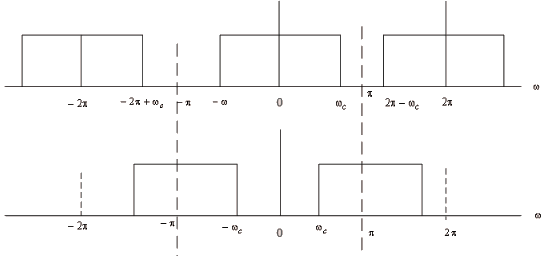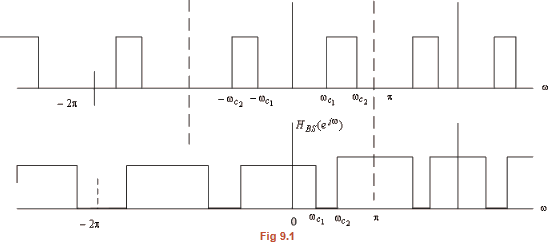The ideal filters have a frequency response that is real and non-negative, in other words, has a zero phase characteristics. A linear phase characteristics introduces a time shift and this causes no distortion in the shape of the signal in the passband.

Since the Fourier transfer of a stable impulse response is continuous function of ω, can not get a stable ideal filter.

Filter specification

Since the frequency response of the realizable filter should be a continuous function, the magnitude response of a lowpass filter is specified with some acceptable tolerance. Moreover, a transition band is specified between the passband and stop band to permit the magnitude to drop off smoothly. Figure (9.2) illustrates this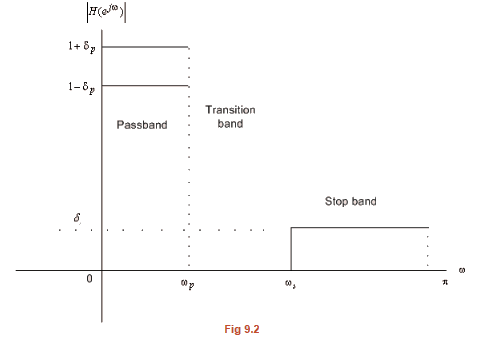In the passband magnitude the frequency response is within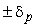of unity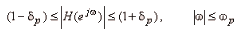In the stopband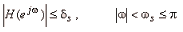The frequencies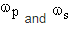are respectively, called the passband edge frequency and the stopband edge frequency. The limits on tolerances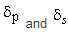are called the peak ripple value. Often the specifications of digital filter are given in terms of the loss function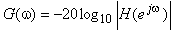, in dB. The loss specification of digital filter are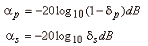Some times the maximum value in the passband is assumed to be unity and the maximum passband deviation, denoted as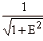is given the minimum value of the magnitude in passband. The maximum stopband magnitude is denoted by. The quantity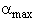is given by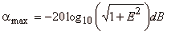These are illustrated in Fig(9.3)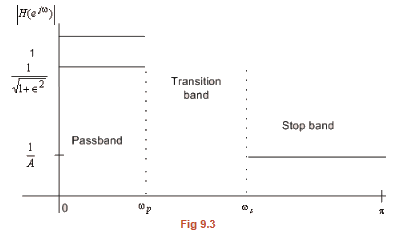If the phase response is not specified, one prefers to use IIR digital filter. In case of an IIR filter design, the most common practice is to convert the digital filter specifications to analog low pass prototype filter specifications, to determine the analog low pass transfer function Ha(s) meeting these specifications, and then to transform it into desired digital filter transfer function. This methods is used for the following reasons:

1. Analog filter approximation techniques are highly advanced.
2. They usually yield closed form solutions.
3. Extensive tables are available for analog-design.
4. Many applications require the digital solutions of analog filters.

The transformations generally have two properties (1) the imaginary axis of the s-plane maps into unit circle of the z-plane and (2) a stable continuous time filter is transformed to a stable discrete time filter

The document Ideal Frequency Selective Filters & Filter Specification Notes | Study Digital Signal Processing - Electrical Engineering (EE) is a part of the Electrical Engineering (EE) Course Digital Signal Processing.
All you need of Electrical Engineering (EE) at this link: Electrical Engineering (EE)Use Code STAYHOME200 and get INR 200 additional OFF

## Digital Signal Processing

3 videos|50 docs|54 tests

Track your progress, build streaks, highlight & save important lessons and more!

,

,

,

,

,

,

,

,

,

,

,

,

,

,

,

,

,

,

,

,

,

;